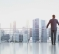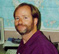## Determine cut-off frequency of filter from transfer function / relationship between sampling frequency and cut-off frequencyStarted by 6 years ago10 replieslatest reply 6 years ago4012 views

Hello!

I've red this Matlab help page on the function "filter" (see below, ) and as an example it said that the filter with transfer function H(z) = 1/(z - 0.9) is a lowpass filter. This made me think: How can I determine the filter characteristic of this filter (e.g. what is the cutoff frequency or the bode diagram in general) and how is this related to sampling frequency? Is the cutoff frequency of this filter different when I pass a signal to it that was sampled with 1kHz vs a signal that was sampled with say 5kHz?

Background: I currently red a paper that proposed some IIR filter for noise reduction of a speech signal. I asked myself weather the proposed filter transfer function is useless anyway because the cutoff frequency will be different depending on the sampling frequency of the input signal.

Kind Regards, Alon

[ - ]Let's keep it simple and first assume your sample rate is unity (1 sample per second, for example).  Frequency scales according to sample rate.  The correct transfer expression for a simple low pass filter is H(z)= a / (1 - (1-a)*z^-1).  Substitute z^-1 = Cos(w)+jSin(w).  When you crunch through the complex arithmetic, you find the 3 db cutoff period is where a = (Cos(360/Period)+Sin(360/Period)-1) / (Cos(360/Period)+Sin(360/Period)) where the angle is in degrees and "Period" is the number of samples per second at the 3 dB point.  By the way, this simplifies to a=5/Period (approximately, over a wide range of values).

Suppose your sample rate is a kilohertz and you want the alpha term for a period of 20.  That is 20 samples per millisecond, or a frequency of 50 Hertz.  Then the alpha term would have a value of 5 / 20 = .25.

[ - ]First, pay attention to the wrong transfer function -- a better one to think with would be H = 0.1/(z - 0.9).

Second, you calculate the gain of the transfer function at any point by calculating $$w = e^{j \theta}$$ where $$\theta$$ is the normalized frequency of a sine wave in radians/sample.  (You can also use $$\theta = \omega T_s = 2 \pi f T_s$$ where $$\omega$$ is the frequency in radians per second, $$T_s$$ is the sample interval, and $$f$$ is the frequency in cycles per second.)

Then you plug $$w$$ into the transfer function in place of $$z$$, and calculate.  Using complex-number arithmetic, if that's not obvious already.

This may help.  Or not, it's old and creaky, but give it a try: http://wescottdesign.com/articles/zTransform/z-tra....

[ - ]Oh, and -- you are correct in your sampling rate worries.  The frequency response of the filter is relative to the signal's frequencies in radians (or cycles) per sample.  So if you have a system that goes ADC -> filter -> DAC and you change the sampling rate without changing the filter characteristics, you'll change the filtering characteristics of the overall system.

This was actually used to advantage in switched-capacitor filters.  They sorta-kinda act like sampled-time systems, so you could design a filter and then tune it just by changing the clock rate.

[ - ]So, assuming I was given a transfer function for a filter which has the right coefficients for, lets say, 12kHz Sampling rate and I plan to use 8kHz... What's the best approach?

A) Upsample my 8kHz Signal to 12kHz to use the filter

B) Redesign the filter (How?) to match the new sampling frequency

What would you guys do?

[ - ]Hi Alon,

Here's some material that may help...  Chapters 14-21 deal with the practical aspects of filter design.  Since you asked about recursive filters, you might want to start with Chapter 19. The filter in your example is commonly called a "single-pole recursive filter", and is discussed at the start of this chapter.

"I asked myself weather the proposed filter transfer function is useless anyway because the cutoff frequency will be different depending on the sampling frequency of the input signal."

Yes, you are correct.

So, assuming I was given a transfer function for a filter which has the right coefficients for, lets say, 12kHz Sampling rate and I plan to use 8kHz... What's the best approach?

I think that is the wrong way to look at the problem.  Instead, choose the cutoff frequency you would like for your particular application, expressed as a fraction of the sampling rate.  You then go through some simple design equations to get the coefficients that implement the filter.

Regards,

Steve

[ - ]Hey Steve thanks for the reply, which was really helpful. I also had a look at the book, it's terrific so thanks for that.

I will look into filter design now. I hope one day I'll be as educated on the subject as you guys! (23 y/o)

[ - ]MATLAB allows you to enter both forward and reverse coeffs.

e.g. freqz(b,a);

b = forward coeffs, i.e. 1 in your case of numerator

a = feedback coeffs i.e. [1, -.9] but you should delete first z to become 1-.9z

you will need to check signs (I can't remember).

as regard sampling frequency. response is relative to Fs of 2 or to FN of 1

It cuts off always as such ratio to sampling speed

[ - ]Thanks for making the effort to reply but unfortunately I did not understand anything that you were trying to say

[ - ]Hah.  My eyeballs passed right over that.  It should (I'm sure, really!) be $$H \left( z \right) = \frac{1}{z - 0.9}$$.  Better would be $$H \left( z \right) = \frac{0.1}{z - 0.9}$$, because then it would have DC gain.